# C++ Constructor: The Complete Guide

0
77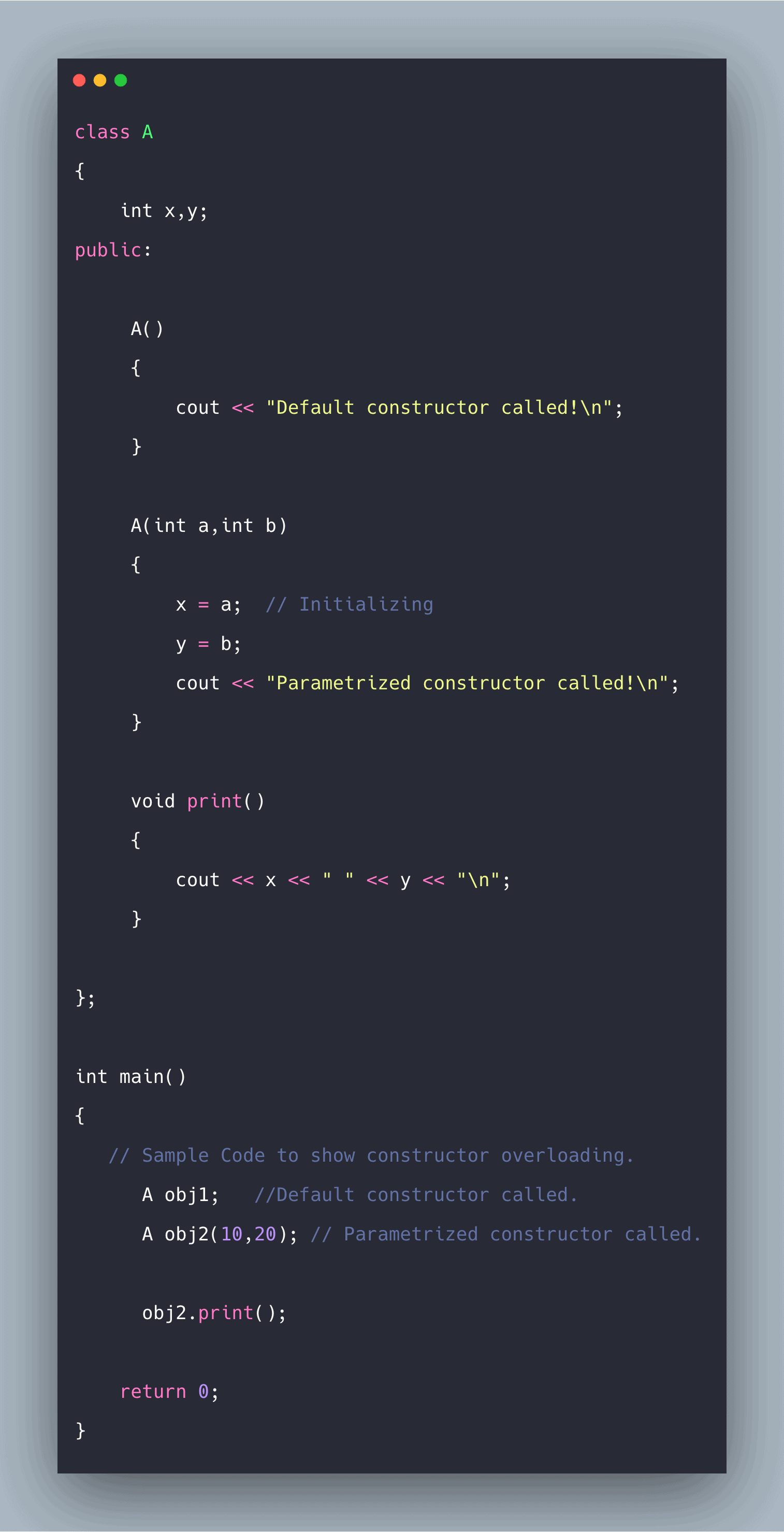C++ constructor is used to initialize the member variables of an instance of a class that is the object of a class. A constructor is a particular type of member function that initializes an object automatically when it is created. The compiler identifies a given member function as a constructor by its name and the return type.

## C++ Constructor

A constructor in C++ is the member function of a class. The constructor has the same name as a class and no return type. Also, the constructor is always public.

But they do differ from actual function in the following ways:

1. The constructor has the same name as the class name.
2. The constructor doesn’t have a return type, unlike other member functions.
3. If there is no explicit definition of a constructor in a class, a default constructor is automatically called.
4. If we do not specify a constructor, the C++ compiler generates a default constructor for us (expects no parameters and has a hollow body).

## How does the constructor works?

Whenever an instance of the class is created, a member function without any return type having the same name as the class is called automatically. Even if this constructor is not defined explicitly, a default constructor is still called.

Since it is a function, it can take arguments and has a function body. So, arguments can be passed to it by explicitly defining custom constructors, and inside its body, arguments passed can be used to initialize the member variables.

## Use of constructors

Constructor, as the name suggests, is usually used to initialize the member variables of the object of a class automatically whenever an object of that class is created. Suppose there is a class Rectangle that stores the length, breadth, and area of the rectangle.

Also, it has some member functions that deal with it. If we want that whenever an object of class Rectangle is created, length, breadth, and area should get initialized automatically. Then, in this case, the constructor is used to initialize the variables.

## Types of constructors in C++

### Default constructor

As the name suggests, a default constructor with no parameters and a hollow body is called if we have not explicitly defined a constructor while defining the class.

To check this, we can explicitly write this default constructor with a print statement inside its body to check that it was called. When an instance or object of a class is created, the default constructor will be called automatically.

The code mentioned below automatically calls the default constructor when the object “obj” of class A is created. It is shown by the print function inside the default constructor, which outputs to the outstream “Default constructor called!”.

See the following default constructor in c++ code.

```#include <iostream>
using namespace std;
class A
{
int x,y;
public:
A()
{
cout << "Default constructor called!";
}
};

int main()
{
// Sample Code to show default constructor
A obj; // making a object of class A
return 0;
}
```

See the below output.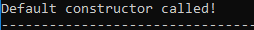### Parameterized constructor

Unlike the default constructor in the parameterized constructor, we can pass as arguments the values to initialize the member variables of an object of a class.

It is the same as a normal function where we pass arguments; the only difference is that it doesn’t have a return type. It is more like function overloading as we can have a different set of constructors for a different set of input parameters, and this is termed constructor overloading.

The parameterized constructor can be called in two ways, namely explicit and implicit.

```class_name obj = class_name(parameter1,parameter2); // Explicit way
class_name obj(parameter 1,parameter 2); //Implicit way
```

Both do the same thing as initializing the member variables of the object of a class.

In the code mentioned below, class A contains two member variables, x, and y. In addition, object obj is created with values 10, and 20 passed to it as arguments.

Here, the implicit calling of the parameterized constructor is called. Then inside the body of the parameterized constructor, ‘x=a’ and ‘y=b’ initialize the object’s member variables.

```#include <iostream>
using namespace std;

class A
{
int x,y;
public:

A()
{
cout << "Default constructor called!";
}

A(int a,int b)
{
x = a;  // Initializing
y = b;
cout << "Parameterized constructor called!\n";
}

void print()
{
cout << x << " " << y << "\n";
}

};

int main()
{
// Sample Code to show default constructor
A obj(10,20); // making a object of class A   -- >Implicit

obj.print();

return 0;
}
```

See the following output.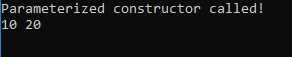### Copy Constructor

It is somewhat different from the other two constructors in that it is used to initialize the member variables of one object by the values of another object of the same class.

Another object of the same class is passed as a reference in the argument, which is then used to initialize the member variables of the concerned object.

It is usually called when a function returns an object or an object is being passed as an argument to a function. It has the following syntax for defining it.

`class_name( const class_name & object_of_same_class );`

When the old object is passed an argument, the values of the member variables of the old object can be explicitly assigned to members of the new object.

In the code mentioned below, two objects, obj, and obj2, have been created.

The first object is just the parameterized constructor used in the above section. obj2  is initialized with the values of the member variables of the object obj, bypassing obj as an argument while creating object obj2. ‘x=old.x’ and ‘y=old.y’ is assigning the values of the old object to a new object.

```#include <iostream>
using namespace std;

class A
{
int x,y;
public:

A()
{
cout << "Default constructor called!";
}
A(int a,int b)
{
cout << "Parameterized Constructor called!\n";
x=a;
y=b;
}
A(const A & old )
{
// old is the old object being passed
x = old.x;  //This object's x to old object's x
y = old.y;

cout << "Copy Constructor called!\n";
}

void print()
{
cout << x << " " << y << "\n";
}

};

int main()
{
// Sample Code to show default constructor
A obj(10,20); // making a object of class A   -- >Implicit
A obj2(obj);  // Copy Constructor called old object 'obj' is passed
obj2.print();

return 0;
}
```

See the below output.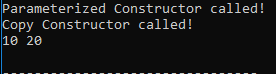So, different constructors can be called based on a different set of arguments passed. However, all the constructors have the same name as the class name.

In the code mentioned below, the different constructor is called based on different argument passed. Class A has two constructors, one without any arguments, that is, the default one, and another with parameters’ a’ and ‘b.

Two objects, obj1 and obj2 are created. In obj1 constructor without arguments is called. The obj2 constructor with parameters is called because parameters’ 10′ and ’20’ have been passed to it.

It sets the member variable of obj2, ‘ x=a’ and ‘y=b.’

See the following code.

```#include <iostream>
using namespace std;

class A
{
int x,y;
public:

A()
{
cout << "Default constructor called!\n";
}

A(int a,int b)
{
x = a;  // Initializing
y = b;
cout << "Parametrized constructor called!\n";
}

void print()
{
cout << x << " " << y << "\n";
}

};

int main()
{
A obj1;   //Default constructor called.
A obj2(10,20); // Parametrized constructor called.

obj2.print();

return 0;
}
```

See the following output.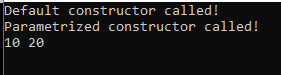That’s it for this tutorial.

This site uses Akismet to reduce spam. Learn how your comment data is processed.